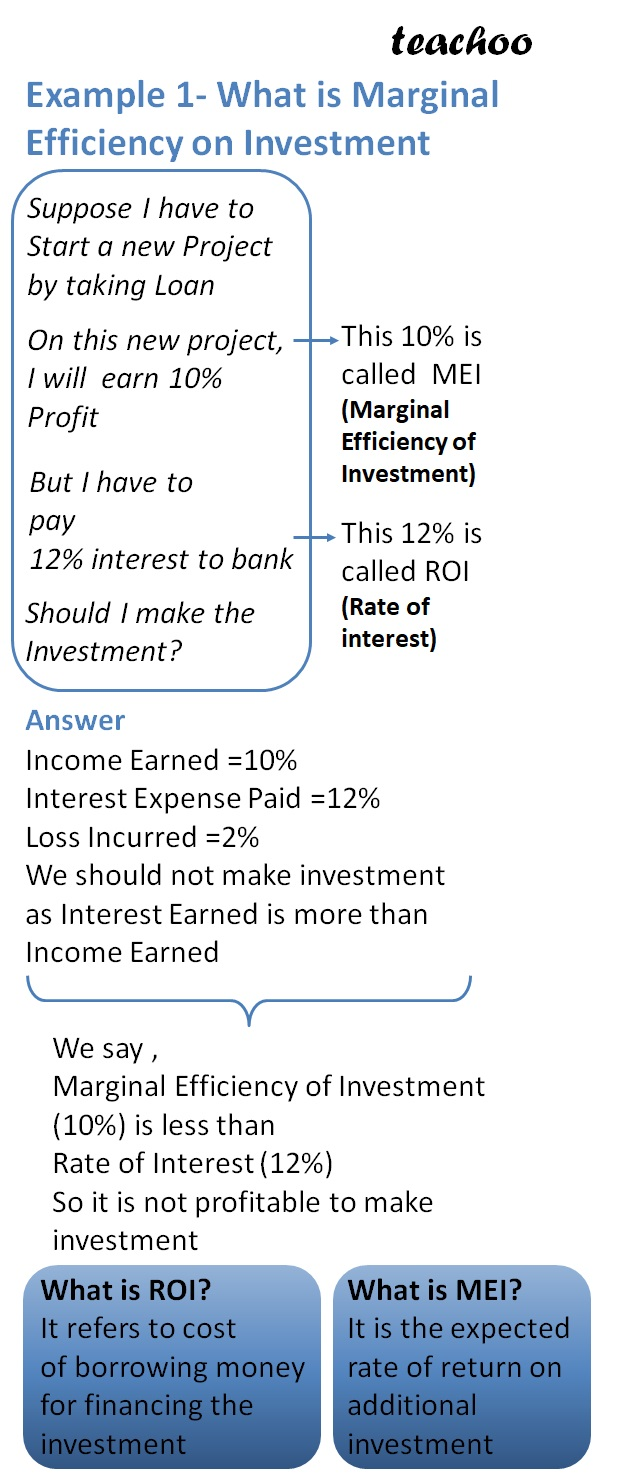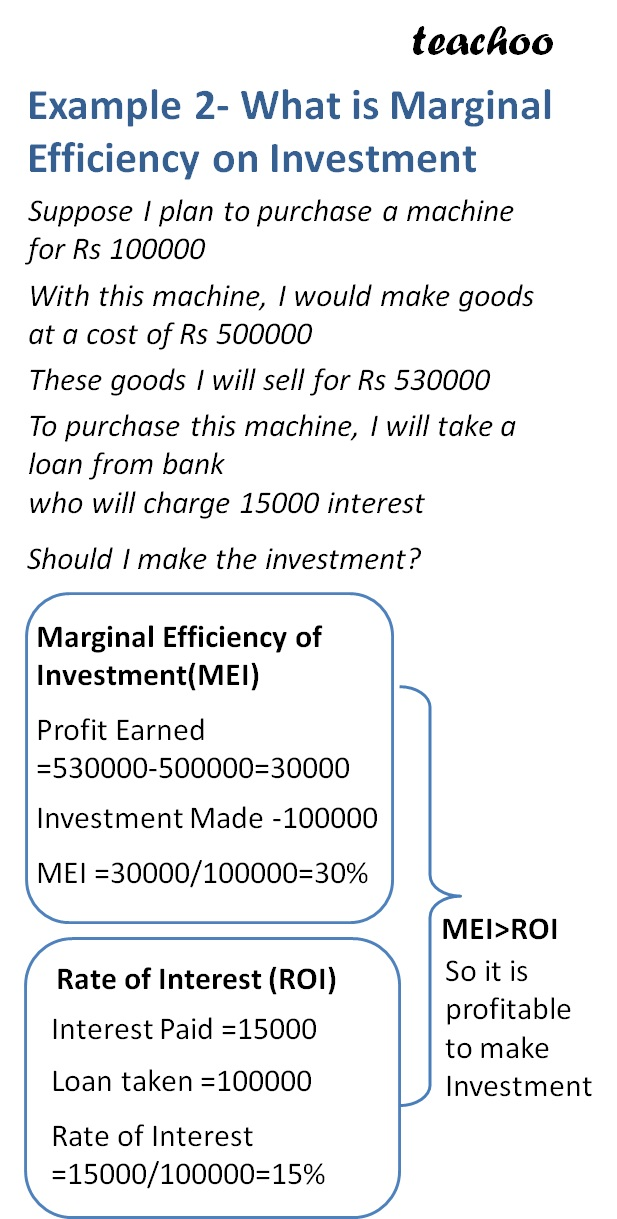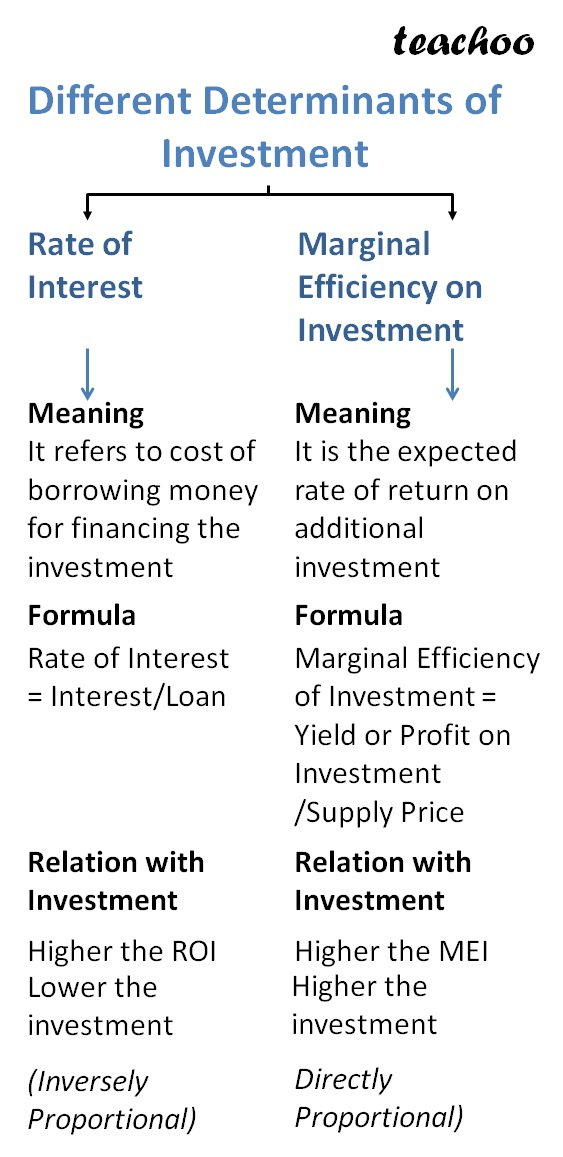Chapter 4 Part 1 - AD,AS and Related Concepts

Economics Class 12
Macroeconomics

## What are Determinants of Investment?

It refers to factors which affect the amount of investment in a project

According to Keynes, there are 2 main determinants of investment

Marginal Efficiency of Investment

Rate of interest

Lets understand them with the help of an exampleExample 1

Suppose I plan to purchase a machine for Rs 100000

With this machine, I would make goods at a cost of Rs 500000

These goods I will sell for Rs 530000

To purchase this machine, I will take a loan from bank who will charge 10000 interest

Should I make the investment?

Rate of Interest on loan = 10000/100000 = 10%

Profit earned from Machine = 530000 - 500000 = 30000

Marginal Efficiency of Investment (Return earned on Machine) = 30000/ 100000 = 30%

Since Marginal Efficiency of Investment is more than Rate of Interest on loan

We should make the investment

What is Marginal Efficiency of Investment? (MEI)

It is the expected rate of return on additional investment

Formula

Marginal Efficiency of Investment = Yield or Profit on Investment/ Supply Price

What is Rate of interest (ROI)?

It refers to cost of borrowing money for financing the investment

How does MEI and ROI Affect Investment?

MEI is directly proportional to investment made

More MEI, More the investment made

ROI is inversely proportional to investment made

Higher the ROI, Lower the investment made and vice versa

### NCERT Questions

No questions in this part

### Other Books

#### Question 1

What are the determinants of Investment?

Explain them.

Get live Maths 1-on-1 Classs - Class 6 to 12

### Transcript

Example 1- What is Marginal Efficiency on Investment Suppose I have to Start a new Project by taking Loan On this new project, I will earn 10% Profit On this new project, I will earn 10% Profit But I have to pay 12% interest to bank Should I make the Investment? This 10% is called MEI (Marginal Efficiency of Investment) This 12% is called ROI (Rate of interest) Answer Income Earned =10% Interest Expense Paid =12% Loss Incurred =2% We should not make investment as Interest Earned is more than Income Earned We say , Marginal Efficiency of Investment (10%) is less than Rate of Interest (12%) So it is not profitable to make investment What is ROI? It refers to cost of borrowing money for financing the investment What is MEI? It is the expected rate of return on additional investment Example 2- What is Marginal Efficiency on Investment Suppose I plan to purchase a machine for Rs 100000 With this machine, I would make goods at a cost of Rs 500000 These goods I will sell for Rs 530000 To purchase this machine, I will take a loan from bank who will charge 15000 interest Should I make the investment? Marginal Efficiency of Investment(MEI) Profit Earned =530000-500000=30000 Investment Made -100000 MEI =30000/100000=30% Rate of Interest (ROI) Interest Paid =15000 Loan taken =100000 Rate of Interest =15000/100000=15% MEI>ROI So it is profitable to make Investment Different Determinants of Investment Rate of Interest Marginal Efficiency on Investment Meaning It refers to cost of borrowing money for financing the investment Meaning It is the expected rate of return on additional investment Rate of Interest = Interest/Loan Marginal Efficiency of Investment = Yield or Profit on Investment /Supply Price Relation with Investment Relation with Investment Higher the ROI Lower the investment (Inversely Proportional) Higher the MEI Higher the investment Directly Proportional)